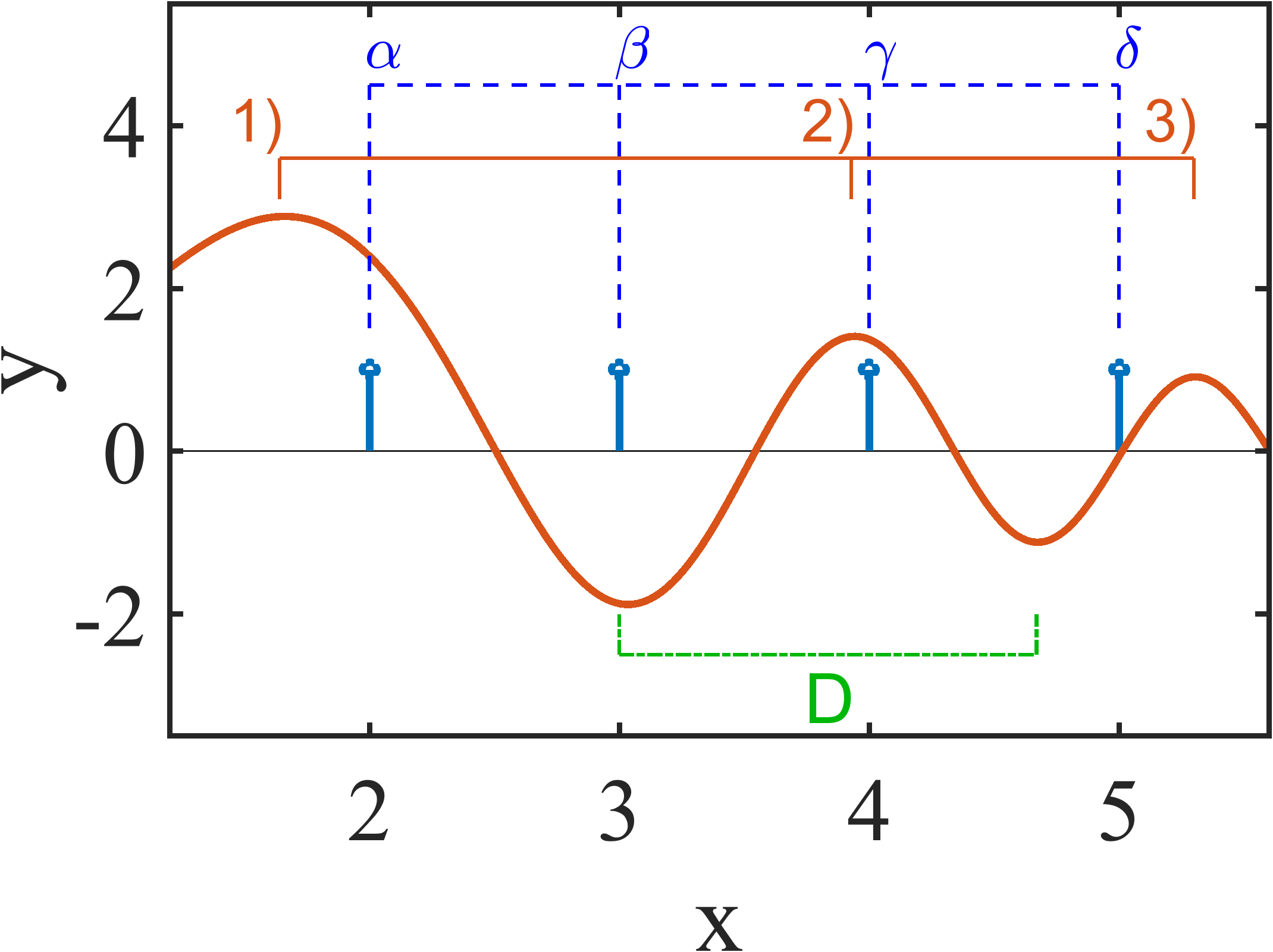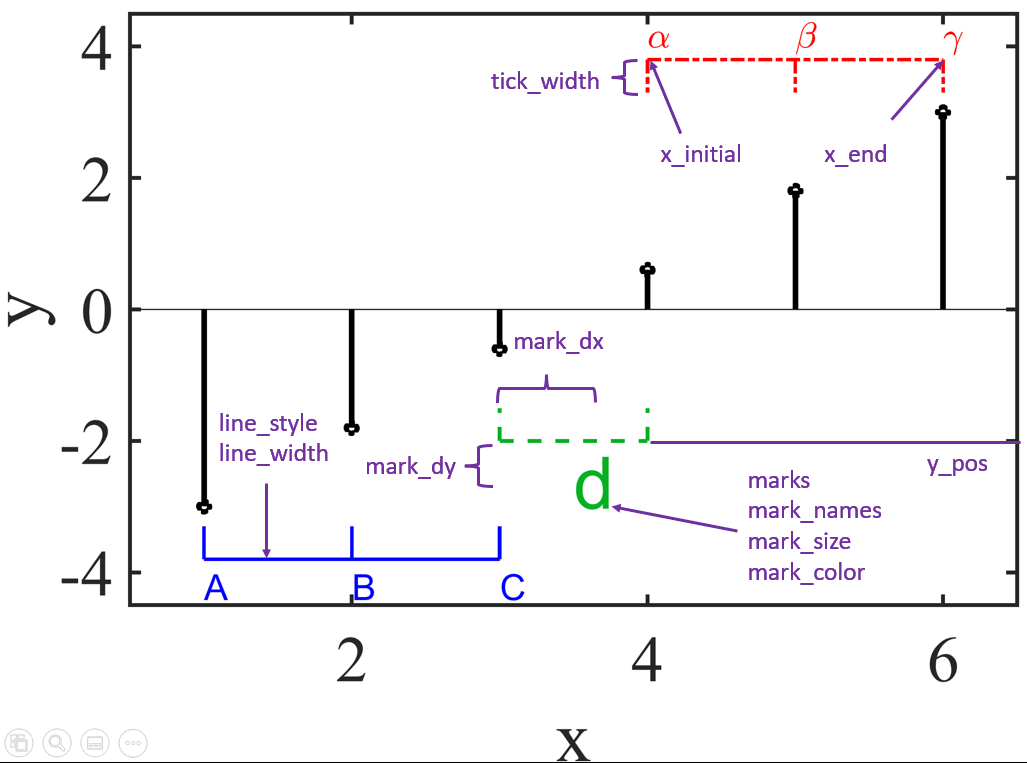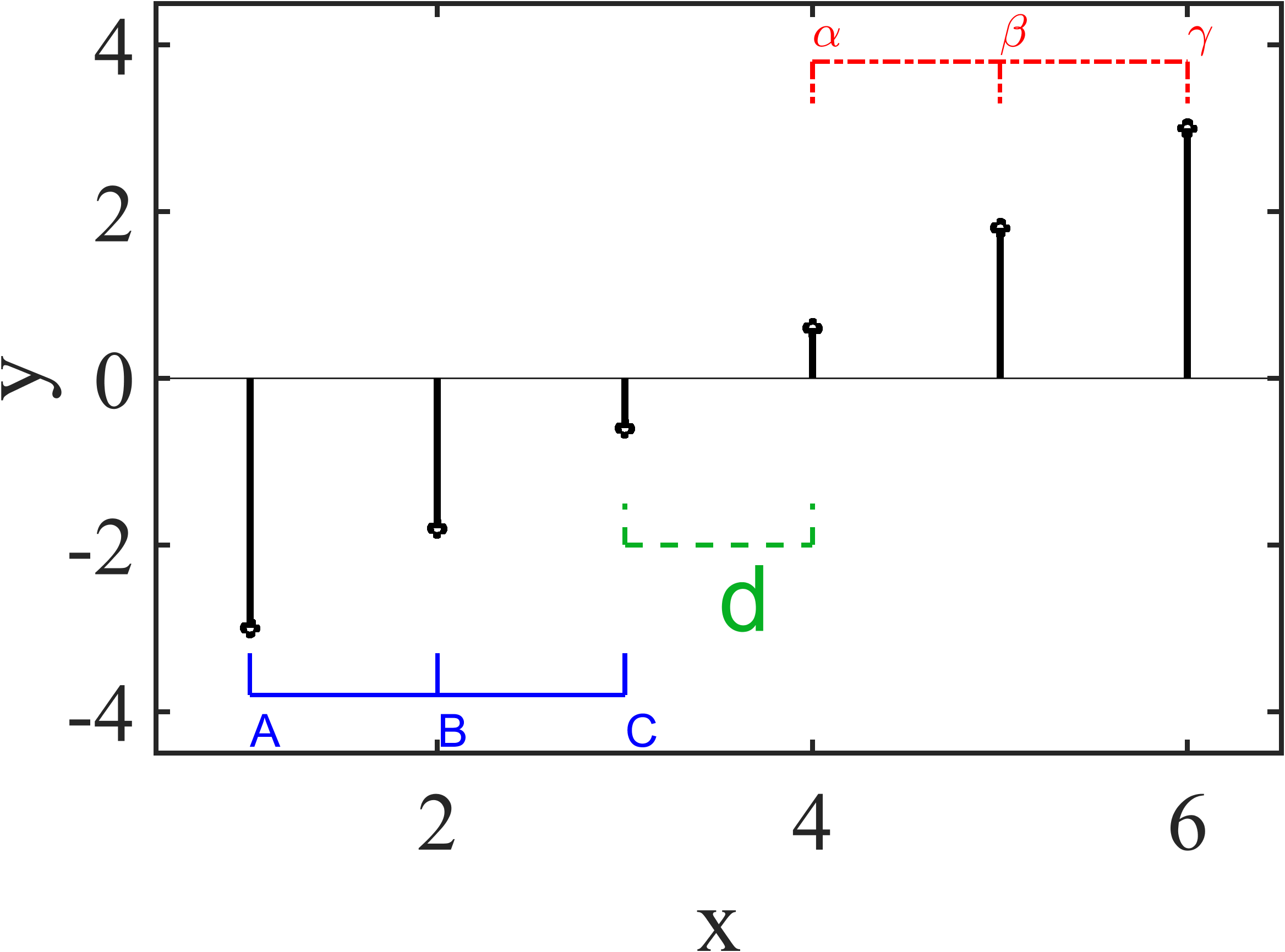LineMarks

version 1.1.1 (1.96 KB) by
Adds "line marks" in order to label and identify constant values or allows to highlight data intervals

Updated 20 Aug 2021

From GitHub

LineMarks

Adds "line marks" in order to label and identify constant values or allows to highlight data intervals on MATLABHow to use this function:

Simply create your MATLAB figure and then execute the function "LineMarks" as follows:

LineMarks(x_initial,x_end,y_pos,marks,mark_names,mark_size,line_style,line_width,tick_width,mark_color,mark_dx,mark_dy)

where (see image below):

x_initial: Initial position for common horizontal line

x_end: Final position for common horizontal line

y_pos: Vertical position for common horizontal line

marks: Float (int or double) array with all the horizontal values for the vertical ticks

mark_names: Cell array with the labels for each mark (use '' for empty)

mark_size: Markers size (font size)

line_style: Line style for all the lines ('-' solid line, '--' dashed line, ':' dotted line, '-.' dash-dot line)

line_width: Line width for all the lines

tick_width: Vertical tick width

mark_color: Color for both markers and lines

mark_dx: Horizontal spacing between lines and labels

mark_dy: Vertical spacing between lines and labelsHow to install this function:

1. This code was written using MATLAB (version 2021a). Download MATLAB on your computer or try MATLAB online
3. Once downloaded, copy these function files to the MATLAB parent directory. On Windows, this is typically: C:\Users\YourUserName\Documents\MATLAB
4. That's it! You should be able to use this function

Example:

y = linspace(-3,3,6); stem(y,'LineWidth',3,'Color','k'); % Creating and plotting some data

xlim([0.5 6.5]); ylim([-4.5 4.5]);

LineMarks(4,6,3.8,[4,5,6],{'\alpha','\beta','\gamma'},20,'-.',2,0.5,'r',0,0.4) % LineMarks #1 (red)

LineMarks(1,3,-3.8,[1,2,3],{'A','B','C'},20,'-',2,-0.5,'b',0,-0.4) % LineMarks #2 (blue)

LineMarks(3,4,-2,[3,4],{'d',''},40,'--',2,-0.5,'#07b023',0.5,-0.6) % LineMarks #3 (green)MATLAB File Exchange:Cite As

Sergio Yanez-Pagans (2022). LineMarks (https://github.com/sergioyapa/LineMarks/releases/tag/1.1.1), GitHub. Retrieved .

MATLAB Release Compatibility
Created with R2021a
Compatible with any release
Platform Compatibility
Windows macOS Linux